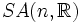# Space group

## Definition

A space group on$\R^n$, also called a crystallographic group, is a subgroup of the group of Euclidean motions that contains some lattice in$\R^n$ as a subgroup, and is contained within the automorphism group of the lattice. In other words, it is a subgroup of the automorphism group of the lattice that contains all the translations by elements of the lattice itself.

Equivalently, it can be defined as a subgroup of the group of Euclidean motions that contains a lattice of translations as a normal subgroup.

### Equivalence notion

Two space groups on$\R^n$ are considered equivalent in the sense of being of the same affine space group type if they are conjugate by some element in the general affine group$GA(n,\R)$.

They are considered equivalent in the sense of being of the same crystallographic space group type if they are conjugate by some element in the special affine group$SA(n,\R)$.

The Beiberbach theorem states that any two space groups that are isomorphic are of the same affine space group type.

## Related notions

### Crystallographic point group

The crystallographic point group of a crystallographic space group is its quotient by the lattice. This group can be identified with a finite subgroup of the orthogonal group$O(n,\R)$. The point group may not completely determine the space group.

### Bravais lattice

The Bravais lattice for a space group is simply its underlying lattice. The term Bravais lattice is typically used when we are considering lattices with respect to the structure of the space group of all their automorphisms.

## Classification

### In one dimension

In one dimension, there are only two space groups: the infinite cyclic group (acting by translations) and the infinite dihedral group (with an infinite cyclic group acting by translations and an element outside acting by reflection). The classification upto affine space group type coincides with the classification upto crystallographic space group type.

### In two dimensions

Further information: Classification of wallpaper groups

The space groups in two dimensions are termed wallpaper groups. There are seventeen wallpaper groups, both upto affine space group type and upto crystallographic space group type. These arise from five kinds of Bravais lattices: the two most symmetric ones are the hexagonal lattice and the square lattice, while the other three are oblique, rectangular, and centered rectangular. Note that every space group is contained in either the space group corresponding to the hexagonal lattice or the space group corresponding to the square lattice.

### In three dimensions

Further information: Classification of three-dimensional space groups

There are 230 crystallographic space group types and 219 affine space group types (11 of the affine space group types differ from their mirror reflections). These arise from 14 kinds of Bravais lattices, and there are 32 crystallographic point groups.